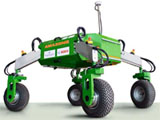## Biological Systems Engineering## Biological Systems Engineering: Papers and Publications

6-1978

#### Citation

TRANSACTIONS of the ASAE (Vol. 21, No.6, pp. 1181, 1182,1183, 1184, 1978)

Copyright 1978 American Society of Agricultural Engineers

#### Abstract

Liquid manure tank wagons were evaluated on a cost basis to determine the optimum size for a given livestock operation. Also the economics of adding a liquid injector to the tank wagon was studied for profitability.

INTRODUCTION

The proper selection of a liquid manure tank wagon is a problem faced by many progressive livestock producers with liquid manure systems. Selection of a manure tank wagon is usually based on the farmer's or dealer's opinion of what size is right rather than on a logical analysis including an economic analysis of the costs involved. This paper provides guidelines for selecting the proper size tank wagon for a given situation based on a least cost approach. Additionally, the economics of liquid manure injection is presented.

EXPLANATION OF THE PROBLEM

In order to select the proper size tank wagon, an economic comparison must be made. This comparison can be based upon the annual costs of using machinery of different sizes. Hunt (1977) gave the following equation for finding the approximate cost of a machine.

ASSUMPTIONS AND PROCEDURE

To use Hunt's equation for approximate annual costs for manure tank wagons requires some assumptions. The term cA/SWe represents hours of field use by a machine. Hours of tanker use will depend on the quantity of manure to be spread, on the tank capacity, and on the distance traveled to and from the disposal area. An assumption of 0.3-h round trip travel time, which includes loading and unloading time, was made. Travel time multiplied by the number of trips required equals the total hours of annual machine use. The number of trips required is determined by dividing the quantity of liquid manure to be hauled annually by the wagon capacity. Values of other constants in equation  can be found in Table 1.

The purchase price of manure tank wagons can be expressed as a function of their capacity in liters. The purchase price per liter is not constant but varies according to capacity. Generally, the tanker price per liter decreases as the size increases with the form of the equation being.

COinS